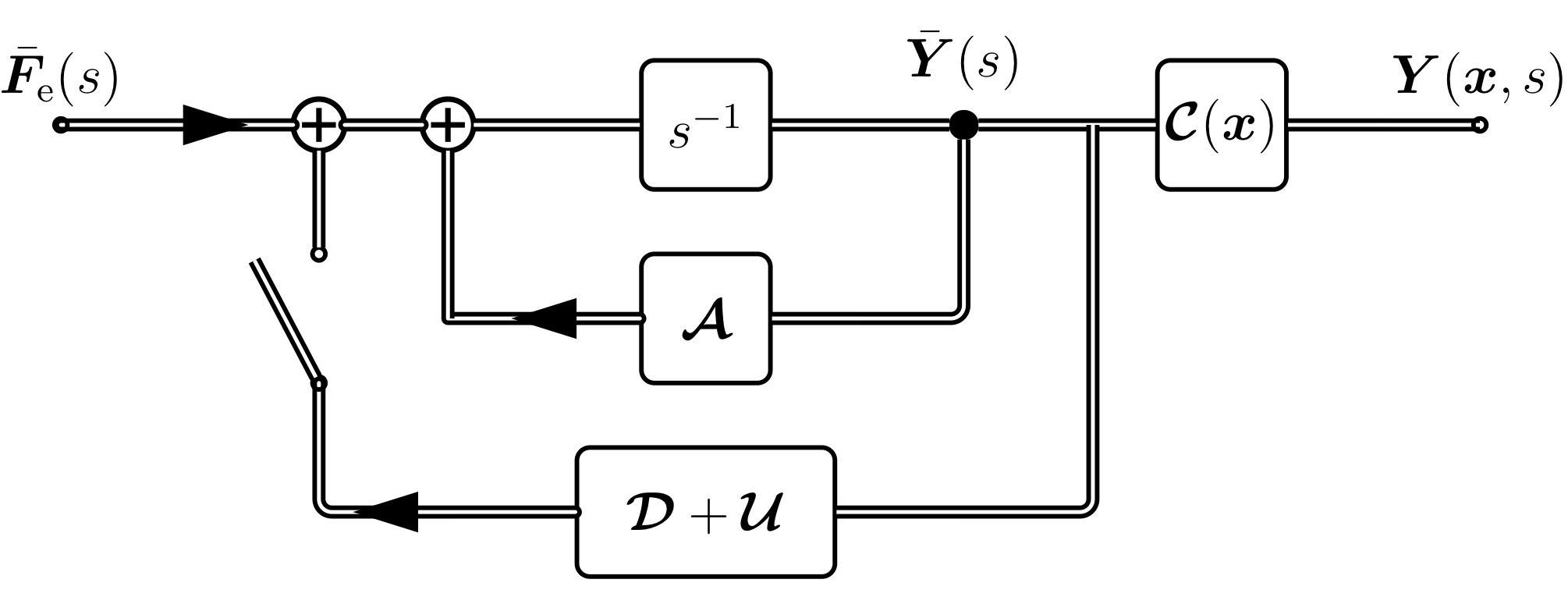# Feedback Structures for a Transfer Function Model of a Circular Vibrating Membrane### Abstract

The attachment of feedback loops to physical or musical systems enables a large variety of possibilities for the modification of the system behavior. Feedback loops may enrich the echo density of feedback delay networks (FDN), or enable the realization of complex boundary conditions in physical simulation models for sound synthesis. Inspired by control theory, a general feedback loop is attached to a model of a vibrating membrane. The membrane model is based on the modal expansion of an initial-boundary value problem formulated in a state-space description. The possibilities of the attached feedback loop are shown by three examples, namely by the introduction of additional mode wise damping; modulation and damping inspired by FDN feedback loops; time-varying modification of the system behavior.

Publication
Proc. IEEE Workshop Applicat. Signal Process. Audio Acoust. (WASPAA)

### Block DiagramFigure 1: State-space description of the 2D circular membrane in the frequency domain (switch open $\widehat{=}$ open loop system) and the attached feedback loop (switch closed $\widehat{=}$ closed loop system).

### Audio Examples

We present various examples of feedback configurations for the proposed system of the circular membrane for sound synthesis.

## Damping Only

In the following, we apply time-invariant damping only in the feedback via the diagonal matrix $\mathcal{D} = \textrm{diag}( \dots, d_{\mu}, \dots )$ and $\mathcal{U} = 0$.

Damping TypeDamping Coefficients
No Damping$d_{\mu} = 0$
Equal Damping$d_{\mu}= -2$
Frequency-dependent Damping$d_{\mu} = -0.001 \cdot k_{n,m}^2/R_0^2$
Tension-like Modulation$d_{\mu} = 0.05j \cdot k_{n,m}^2/R_0^2$

Sound example with various damping feedback loop.

## Time Variation

In the following, we apply time-variant coupling only in the feedback via the matrix $\gamma_\textrm{U}[k] \cdot \mathcal{U}$ with $\gamma_\textrm{U}[k] = \alpha \sin( \beta , k 2\pi T)$, where $\alpha$ denotes the time-variation amplitude and $\beta$ denotes the time-variation frequency in Hertz ($\mathcal{D} = 0$).

Feedback Matrix TypeTime variation Amplitude $\alpha$Time variation Frequency $\beta$
Identity0.000
Random Orthogonal0.0215

Sound example with various strength of time-variation.

## Experimental Configurations

In the following, we apply time-variant coupling only ($\mathcal{D} = 0$) in the feedback via the matrix with similar parameters as above, but more experimental settings.

Feedback Matrix TypeTime variation Amplitude $\alpha$Time variation Frequency $\beta$
Identity0.000